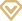# 2015上半年SAT模拟试题：数学

2015-04-17 15:56:00   无忧考网     [ 字体： ] [ 手机版 ] [文档预览 ] [文档下载 ]2015上半年SAT模拟试题：数学

1. Which of the following could be a value of x, in the diagram above?
A. 10
B. 20
C. 40
D. 50
E. any of the above
2. Helpers are needed to prepare for the fete. Each helper can make either 2 large cakes or 35 small cakes per hour. The kitchen is available for 3 hours and 20 large cakes and 700 small cakes are needed. How many helpers are required?
A. 10
B. 15
C. 20
D. 25
E. 30
3. Jo's collection contains US, Indian and British stamps. If the ratio of US to Indian stamps is 5 to 2 and the ratio of Indian to British stamps is 5 to 1, what is the ratio of US to British stamps?
A. 5 : 1
B. 10 : 5
C. 15 : 2
D. 20 : 2
E. 25 : 2
4. A 3 by 4 rectangle is inscribed in circle. What is the circumference of the circle?
A. 2.5π
B. 3π
C. 5π
D. 4π
E. 10π
5. Two sets of 4 consecutive positive integers have exactly one integer in common. The sum of the integers in the set with greater numbers is how much greater than the sum of the integers in the other set?
A. 4
B. 7
C. 8
D. 12
E. it cannot be determined from the information given.

Explanation:
The marked angle, ABC must be more than 90 degrees because it is the external angle of triangle BDC, and must be equal to the sum of angles BDC (90) and DCB. Also ABC is not a straight line and must be less than 180. Therefore 90 < 5x < 180 The only value of x which satisfies this relation is 20.
Explanation:
20 large cakes will require the equivalent of 10 helpers working for one hour. 700 small cakes will require the equivalent of 20 helpers working for one hour. This means if only one hour were available we would need 30 helpers. But since three hours are available we can use 10 helpers.
Explanation:
Indian stamps are common to both ratios. Mul
ltiply both ratios by factors such that the Indian stamps are represented by the same number. US : Indian = 5 : 2, and Indian : British = 5 : 1. Multiply the first by 5, and the second by 2.
Now US : Indian = 25 : 10, and Indian : British = 10 : 2 Hence the two ratios can be combined and US : British = 25 : 2
Explanation:
Draw the diagram. The diagonal of the rectangle is the diameter of the circle. The diagonal is the hypotenuse of a 3,4,5 triangle, and is therefore, 5. Circumference = π.diameter = 5π